# 06-240/Homework Assignment 2

Read sections 1.1 through 1.3 in our textbook, and solve the following problems:

• Problems 3a and 3bcd on page 6, problems 1, 7, 18, 19 and 21 on pages 14-16 and problems 8, 9, 11 and 19 on pages 20-21. You need to submit only the underlined problems.
• Note that the numbers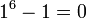$1^6-1=0$,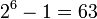$2^6-1=63$,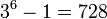$3^6-1=728$,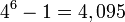$4^6-1=4,095$,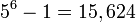$5^6-1=15,624$ and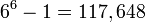$6^6-1=117,648$ are all divisible by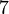$7$. The following four part exercise explains that this is not a coincidence. But first, let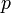$p$ be some odd prime number and let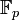${\mathbb F}_p$ be the field with p elements as defined in class.
1. Prove that the product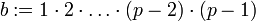$b:=1\cdot 2\cdot\ldots\cdot(p-2)\cdot(p-1)$ is a non-zero element of${\mathbb F}_p$.
2. Let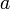$a$ be a non-zero element of${\mathbb F}_p$. Prove that the sets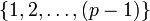$\{1,2,\ldots,(p-1)\}$ and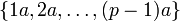$\{1a,2a,\ldots,(p-1)a\}$ are the same (though their elements may be listed here in a different order).
3. With$a$ and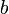$b$ as in the previous two parts, show that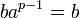$ba^{p-1}=b$ in${\mathbb F}_p$, and therefore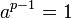$a^{p-1}=1$ in${\mathbb F}_p$.
4. How does this explain the fact that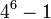$4^6-1$ is divisible by$7$?

You don't need to submit this exercise at all, but you will learn a lot by doing it!

This assignment is due at the tutorials on Thursday September 28. Here and everywhere, neatness counts!! You may be brilliant and you may mean just the right things, but if the teaching assistants will be having hard time deciphering your work they will give up and assume it is wrong.

An email I got -

Date: Mon, 18 Sep 2006 13:53:19 -0300
To: calculusthemusical@gmail.com
Subject: "Calculus: The Musical!"

Greetings!

and students.

I am contacting you from Matheatre, a performance duo originating in
Minneapolis, Minnesota. We are excited to announce that our newest
touring show, "Calculus: The Musical!" is scheduled to perform a limited
engagement in Toronto September 28-30!

"Calculus: The Musical" is a comic "review" of the concepts and history
of calculus. Using musical parodies that span genres from light opera to
hip-hop, the quest for the instantaneous rate of change and the area
under the curve comes to life--through song! Written by a licensed math
teacher, "Calculus: The Musical!" puts the "edge" back in "education!"

"Calculus: The Musical!"
Thursday, September 28, 7pm
Friday, September 29, 7pm
Saturday, September 30, 8:30pm
Unit 102 Theatre
46 Noble Street
(near the intersection of Dufferin and Queen)
Tickets: \$10.00